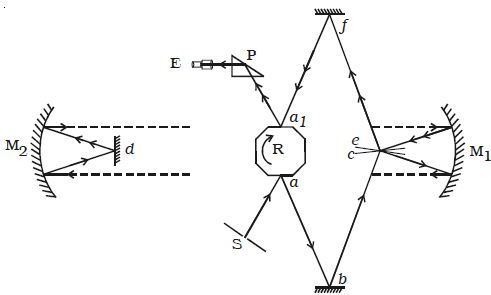# Explain Michelson’s Method

A.A. Michelson, an American physicist, spent many years of his life in measuring the velocity of light and he devised a method in the year 1926 which is considered as accurate.Fig: Michelson’s Method

The experimental set up is shown in Figure. Light from an arc source after passing through a narrow slit S is reflected from one face α of an octagonal mirror R. The ray after reflections at small fixed mirrors b and c is then rendered parallel by a concave mirror M1 placed in the observing station on Mt. Wilson. This parallel beam of light travels a distance of 35 km and falls on another concave mirror M2 placed at Mt. St Antonio, and it is reflected to a plane mirror d placed at the focus of the concave mirror M2. The ray of light from d is rendered parallel after getting reflected by M2 and travels back to the concave mirror M1.

After reflections at M1 and the plane mirrors e and f. the ray falls on the opposite face a1 of the octagonal mirror. The final image which is totally reflected by a total reflecting prism P, is viewed through an eye piece E.

When the octagonal mirror is stationary, the image of the slit is seen through the eye piece. When it is rotated the image disappears. The speed of rotation of R is suitably adjusted so that the image is seen again clearly as when R is stationary. The speed of revolution is measured by stroboscope.

Let D be the distance travelled by light from face α to face al and n be the number of rotations made by R per second.

The time taken by R to rotate through 45° or 1/8 of a rotation = 1/8n

During this time interval, the distance travelled by the light = D

so, The velocity of light c = [Distance travelled/ Time taken] = [D/(1/8n)] = 8nD.

In general, if the number of faces in the rotating mirror is N, the velocity of light = NnD.

The velocity of light determined by him is 2.99797 x 108 m s-1.